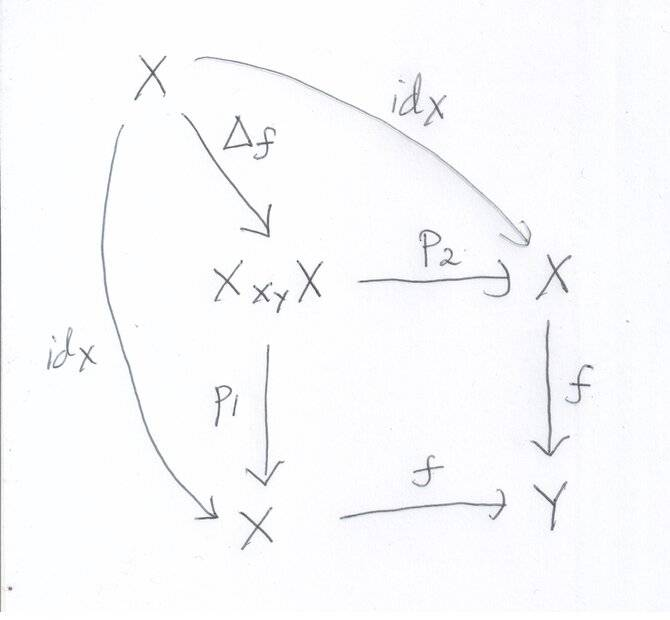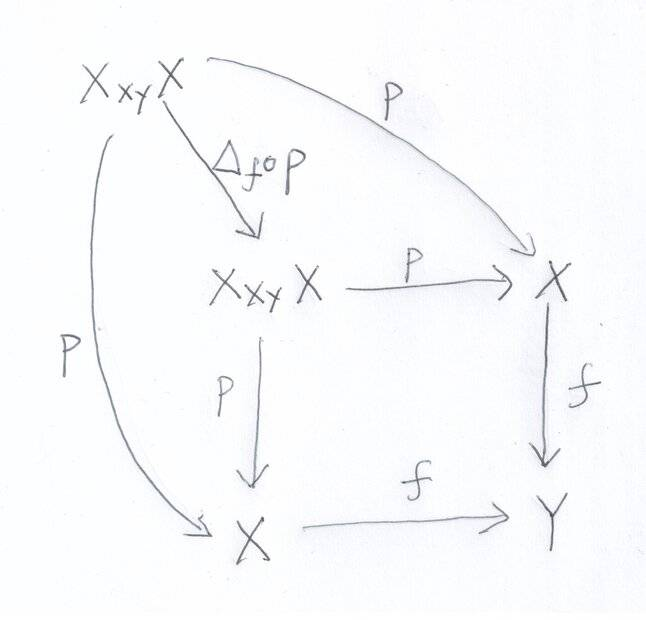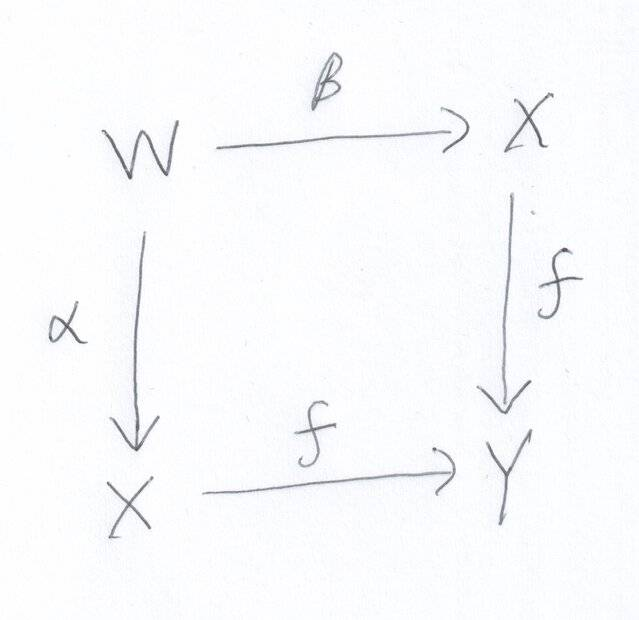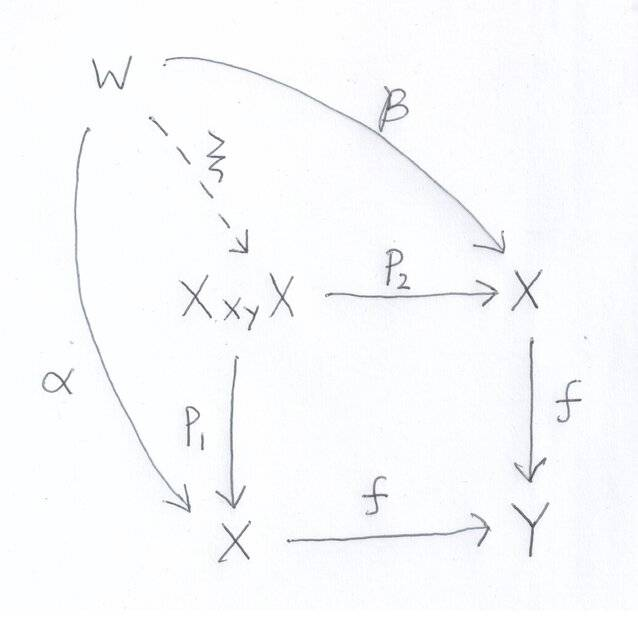# In a Category with Fibered Products

• POTW
• Euge

#### Euge

Gold Member
MHB
POTW Director
Let ##\mathscr{C}## be a category in which fibered products exist. If ##f : X \to Y## is a morphism in ##\mathscr{C}##, prove that ##f## is a monomorphism if and only if the diagonal morphism ##\Delta_f : X \to X\times_Y X## is an isomorphism.

•topsquark, anemone, malawi_glenn and 2 others

I have actually done some category theory, so I might have a go at the problem.

"Only if" part:

We assume ##f## is monomorphic.

(i) Let ##p_1 : X \times_Y X \rightarrow X## and ##p_2 : X \times_Y X \rightarrow X## be the canonical projection morphisms. See figure A. We have ##f \circ p_1 = f \circ p_2##. As ##f## is monomorphic we have ##p_1 = p_2 = p##. Thus there is a unique ##p## such that ##p \circ \Delta_f = \text{id}_X##.Figure A

(ii) See figure B. By definition of a fibered product, ##\Delta_f \circ p## is uniquely determined. By (i) we have ##p \circ \Delta_f \circ p = p##, meaning that by the uniqueness of ##\Delta_f \circ p##, we have ##\Delta_f \circ p = \text{id}_{X \times_Y X}##.Figure B

"If" part:

We assume that ##\Delta_f## is an isomorphism.

We wish to prove that if ##f \circ \alpha = f \circ \beta## (i.e. figure C) then ##\alpha = \beta##, when ##\Delta_f## is an isomorphism.Figure C

First note: that as ##\Delta_f## is an isomorphism it has a unique inverse morphism, and as such ##p_1 = p_2 = p##.

See figure D. By definition of a fibered product, there is a unique morphism ##\xi : W \rightarrow X \times_Y X## such that ##\alpha = p_1 \circ \xi## and ##\beta = p_2 \circ \xi##. As ##p_1 = p_2 = p##, we have ##\alpha = p \circ \xi = \beta##.Figure D

•topsquark, Greg Bernhardt, malawi_glenn and 1 other person
Thanks @julian for participating. Your solution is correct! So the postgraduate problems are not all beyond you.•julian
Thanks @julian for participating. Your solution is correct! So the postgraduate problems are not all beyond you.I guess I have read some graduate maths in my time. But reading graduate maths and doing problems are two different things. This gives me some confidence in at least trying the problems. I may not always solve them but I would probably learn some more maths in attempting doing so, which I guess is one of the main reasons for the problems!

This has given me incentive to go back and better understand category theory!

•PhDeezNutz, topsquark, Greg Bernhardt and 1 other person
Off topic. But any ideas for beginner books on cathegory theory and reasonable requirnents?

You can start with Steve Awodey's Category Theory and/or Emily Riehl's Category Theory in Context.

It would help to have some background knowledge in abstract algebra, at least at the undergraduate level. A topology background is also useful, but in my view not required.

Last edited:
•Ackbach and malawi_glenn